table div table+table div table{width:100%;padding:0}table div table+table div table img{width:96.23%;padding:0;float:none}table div table+table div table td{width:100%;padding:0 1.88% 18px}/* styles */## "Life is a flower of which love is the honey." Victor Hugo

 table div table+table+table+table div table{width:100%;padding:0}table div table+table+table+table div table img{width:96.23%;padding:0;float:none}table div table+table+table+table div table td{width:100%;padding:0 1.88% 18px}/* styles */table div table+table+table+table+table div table{width:100%;padding:0}table div table+table+table+table+table div table img{width:96.23%;padding:0;float:none}table div table+table+table+table+table div table td{width:100%;padding:0 1.88% 18px}/* styles */## Jazz, Honey Wine and heART inspired valentines!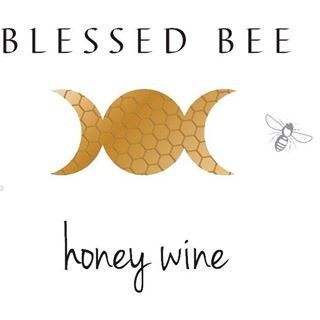## Wine tastings ~ Organic, premium MEAD wine with 100% honey, herbs and natural flavors.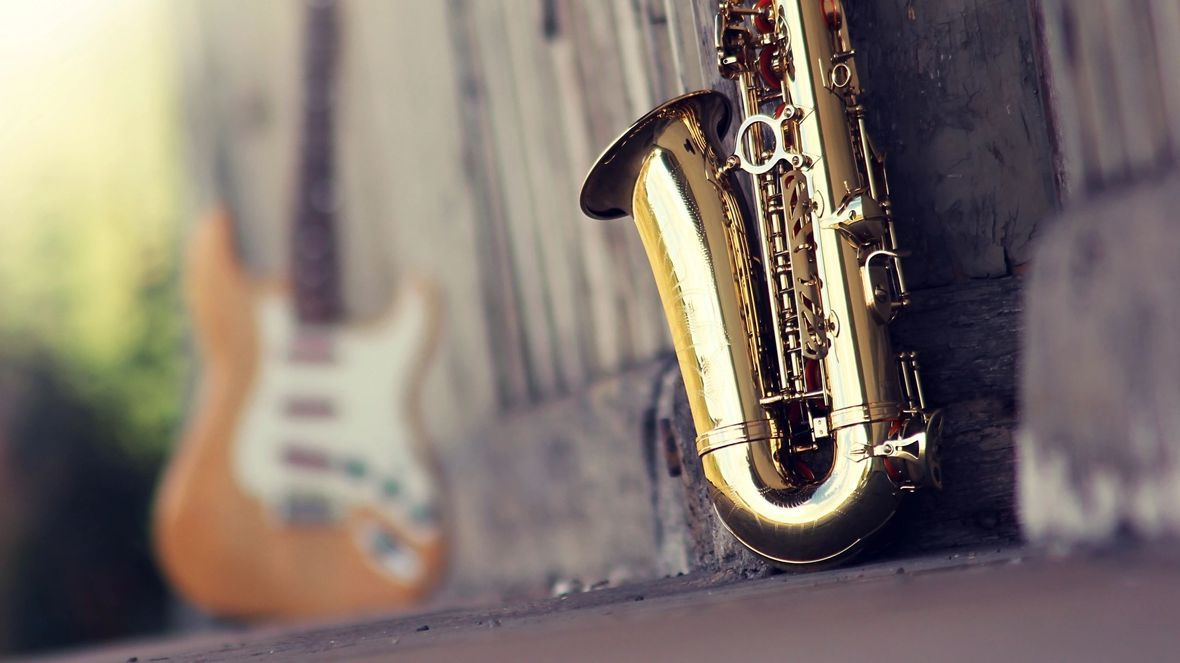## Joe Mantheiy & Paradox Jazz will be playing at the gallery!

 table div table+table+table+table+table+table+table+table+table+table+table+table div table{width:100%;padding:0}table div table+table+table+table+table+table+table+table+table+table+table+table div table img{width:96.23%;padding:0;float:none}table div table+table+table+table+table+table+table+table+table+table+table+table div table td{width:100%;padding:0 1.88% 18px}/* styles */## Featuring the encaustic works of Lyn Belise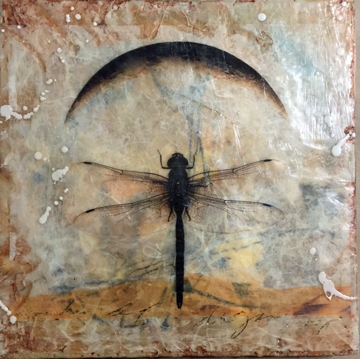Lyn Belisle, Dragonfly, encaustic, 10"x10", \$150.
 /* styles */ What is Encaustic Painting? In fine art, the word "encaustic" describes both the paint and painting technique which uses hot beeswax to bind colour pigments and to facilitate their application to a surface. An invention of Greek art, its name derives from the Greek word meaning "burnt in". The paint is applied to the painting surface (usually a wooden panel, or a wall), after which it is reheated to fuse the paint into a uniform enamel-like finish, devoid of all brushmarks. Ancient artists applied the paint using brushes and spatulas to create the image. On completion, they applied a flaming torch to the painting's surface to reheat the wax, causing it to meld permanently with the pigments and with the panel.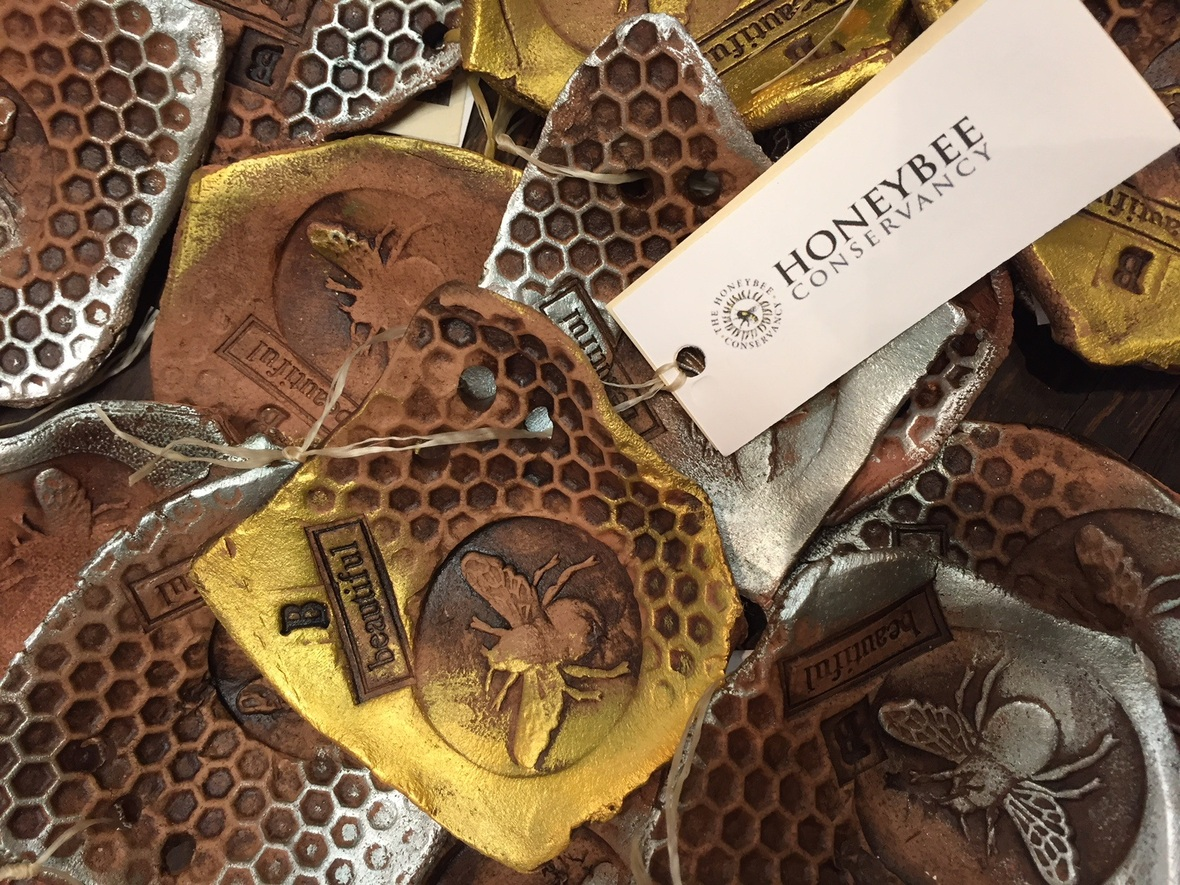/* styles */ Cradle your soap, your tea bag, your rings...in this little handbuilt earthenware. Celebrate everyday beauty! As an artist who uses beeswax in her work, Lyn Belisle, is concerned about the honeybees and the problem of Colony Collapse Disorder. She supports the work of the Honeybee Conservance by donating \$1 from each sale of bee dish to the research fund. Please visit their excellent informational website, www.thehoneybeeconservancy.org
 table div table+table+table+table+table+table+table+table+table+table+table+table+table+table+table+table+table+table div table{width:100%;padding:0}table div table+table+table+table+table+table+table+table+table+table+table+table+table+table+table+table+table+table div table img{width:96.23%;padding:0;float:none}table div table+table+table+table+table+table+table+table+table+table+table+table+table+table+table+table+table+table div table td{width:100%;padding:0 1.88% 18px}/* styles */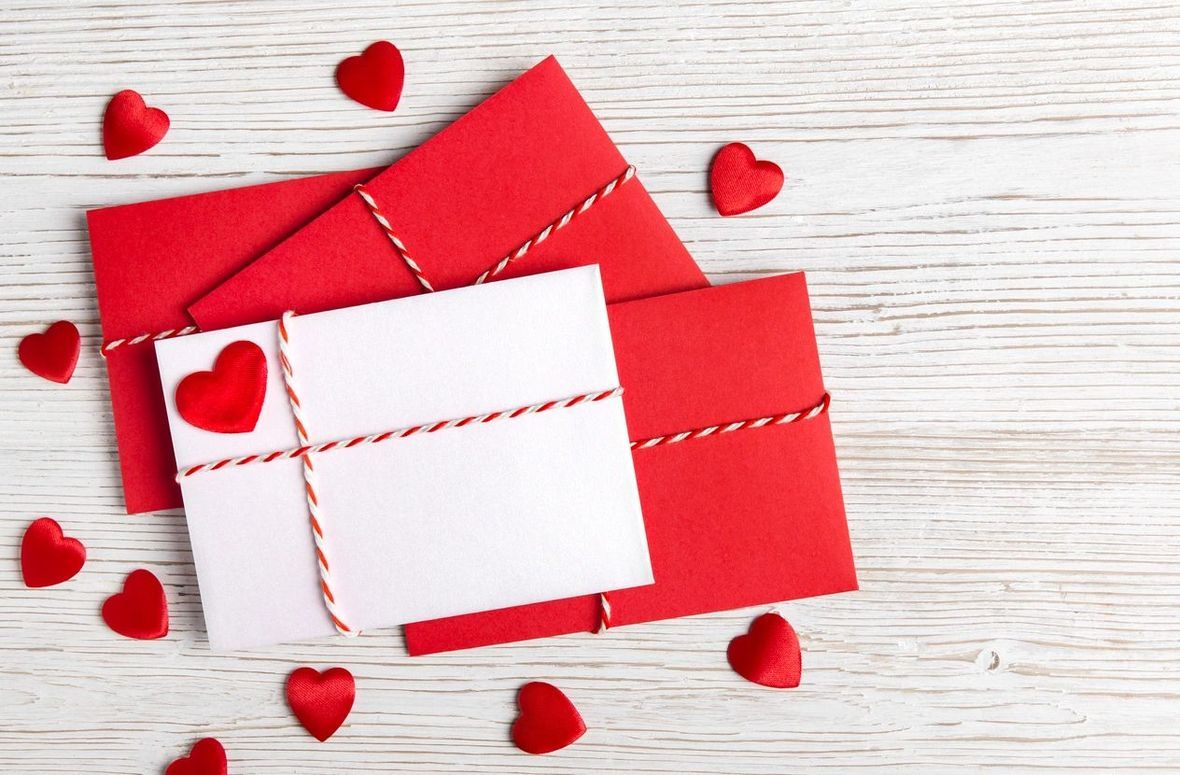## Monday, February 5th, 10:30am to 2pm

 /* styles */ All materials are provided. Class is limited to 12 people - perfect for your book club, social club or Bible study group! RSVP by Email info@martastaffordfineart.com. Lunch will not be provided, but we will break for lunch and visit one of our local Main Street eateries. Cost \$40. per person.
 table div table+table+table+table+table+table+table+table+table+table+table+table+table+table+table+table+table+table+table+table+table+table+table+table div table{width:100%;padding:0}table div table+table+table+table+table+table+table+table+table+table+table+table+table+table+table+table+table+table+table+table+table+table+table+table div table img{width:96.23%;padding:0;float:none}table div table+table+table+table+table+table+table+table+table+table+table+table+table+table+table+table+table+table+table+table+table+table+table+table div table td{width:100%;padding:0 1.88% 18px}/* styles */## Linda Draper Jewelry Designs

 /* styles */ 35" long strand of recycled red glass beads with natural rock crystal accents, the clear white beads ( cubes and "cylinders") are rock crystal or quartz with small glass crystal spacers. The clasp and ear wires are Sterling Silver. \$170.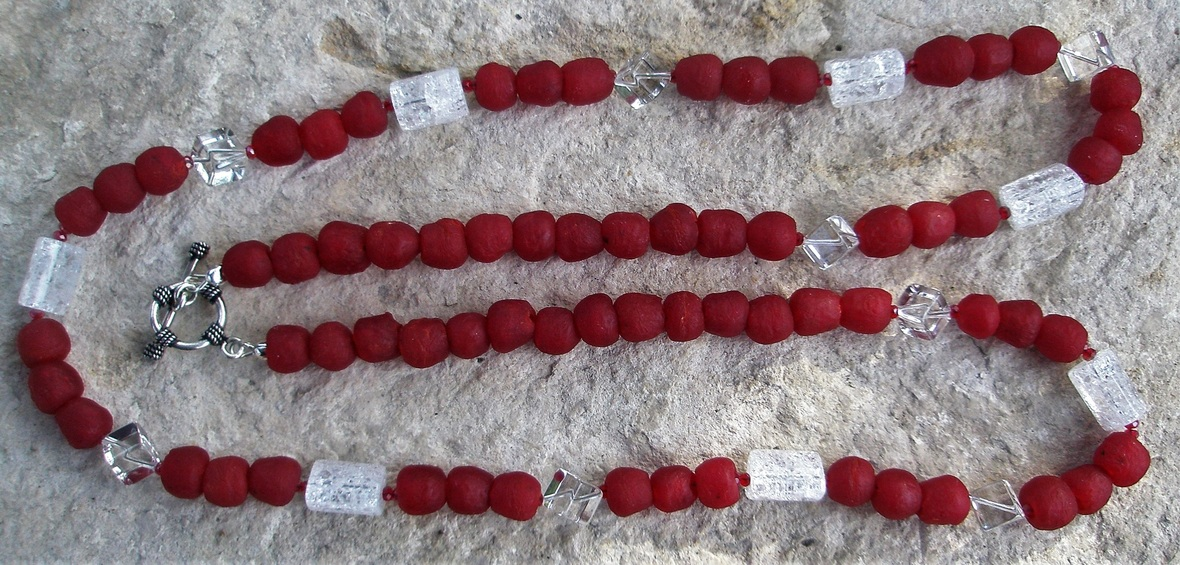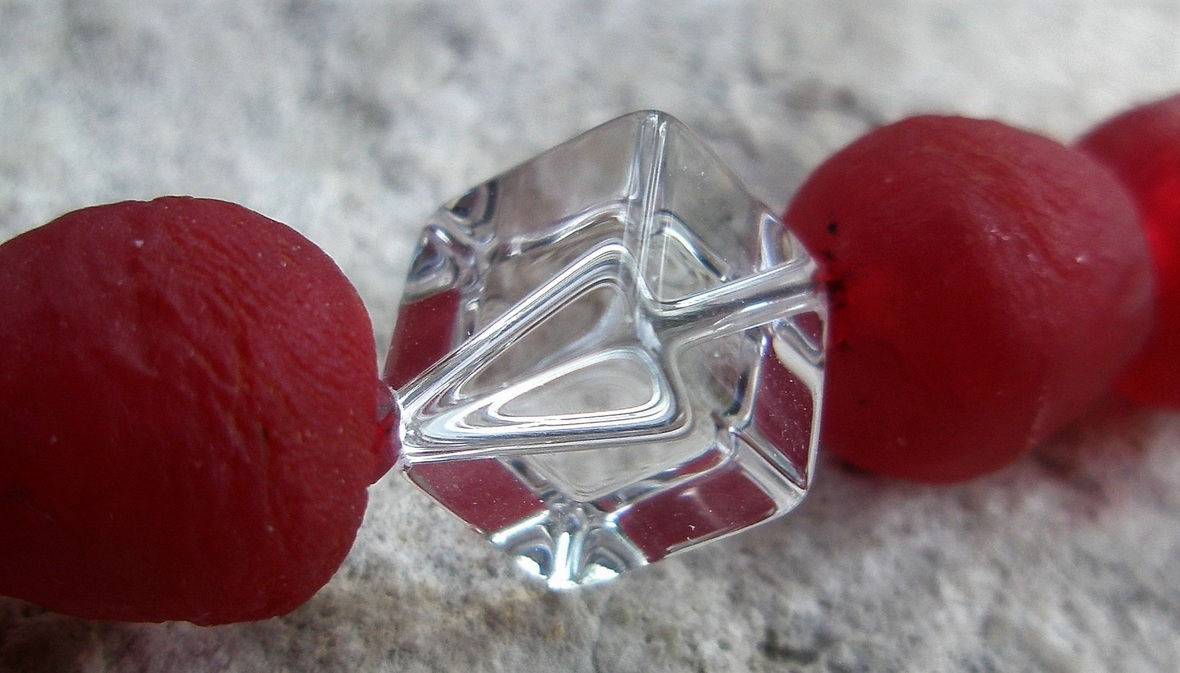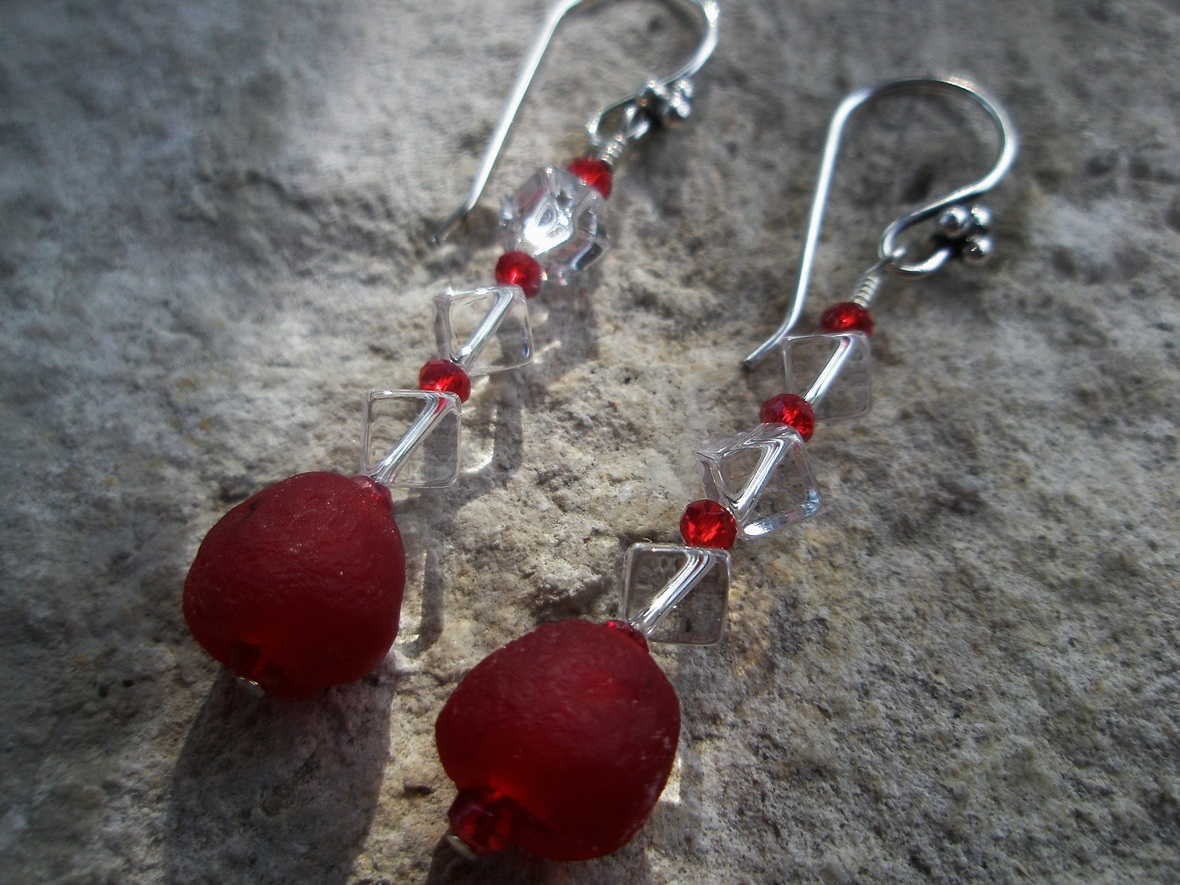table div table+table+table+table+table+table+table+table+table+table+table+table+table+table+table+table+table+table+table+table+table+table+table+table+table+table+table+table+table+table div table{width:100%;padding:0}table div table+table+table+table+table+table+table+table+table+table+table+table+table+table+table+table+table+table+table+table+table+table+table+table+table+table+table+table+table+table div table img{width:96.23%;padding:0;float:none}table div table+table+table+table+table+table+table+table+table+table+table+table+table+table+table+table+table+table+table+table+table+table+table+table+table+table+table+table+table+table div table td{width:100%;padding:0 1.88% 18px}/* styles *//* styles */ Tribal style triple strand. Vintage African Trade Beads The tab and the big black and white roundish beads are batik dyed bone. The large dark fluted beads are hand carved ebony wood, the small dark fluted ones are called "dog tooth" beads, and the white beads are called Masai beads. The brass beads are hand cast. The cllasp and ear wires are Sterling Silver. \$200.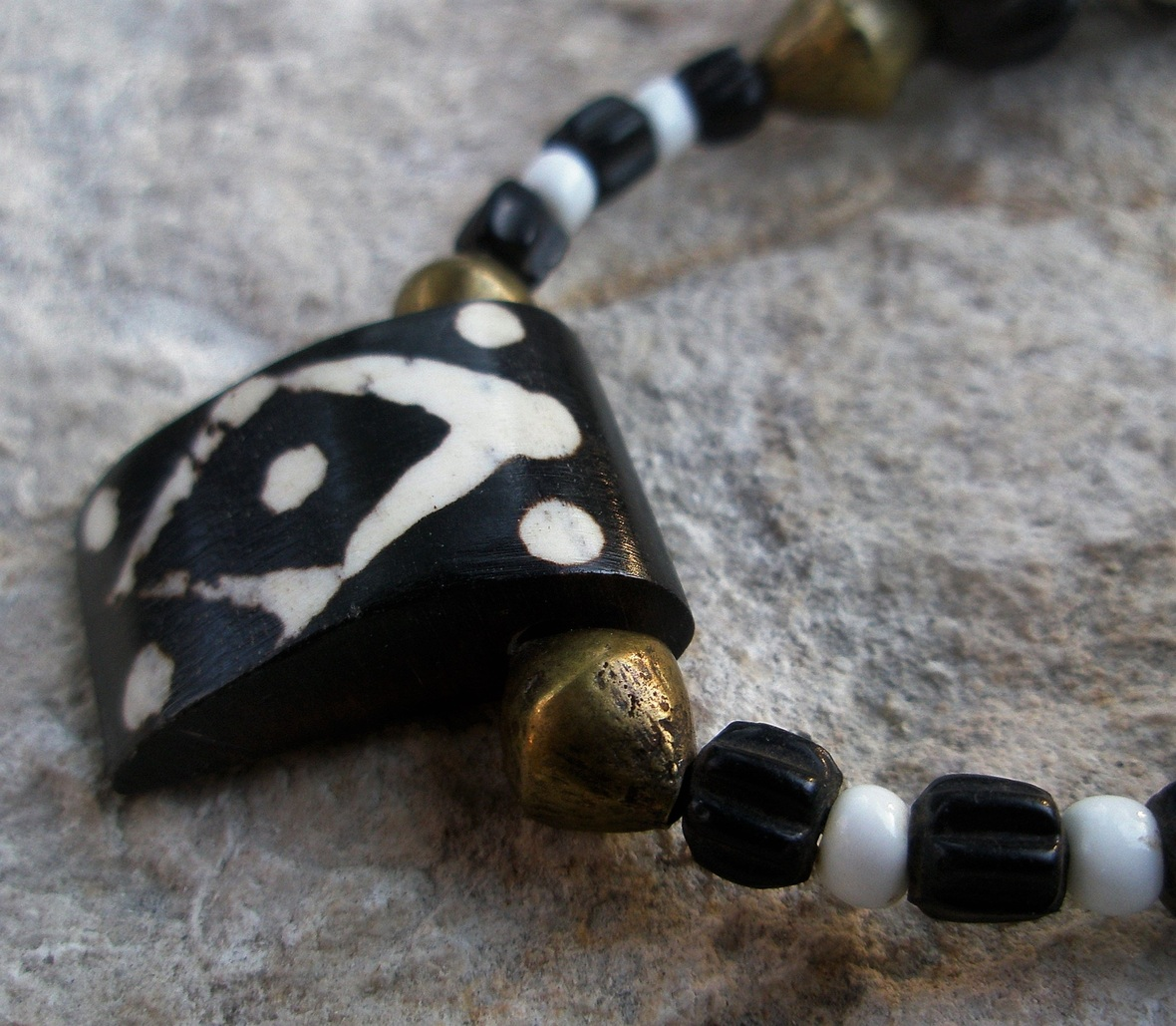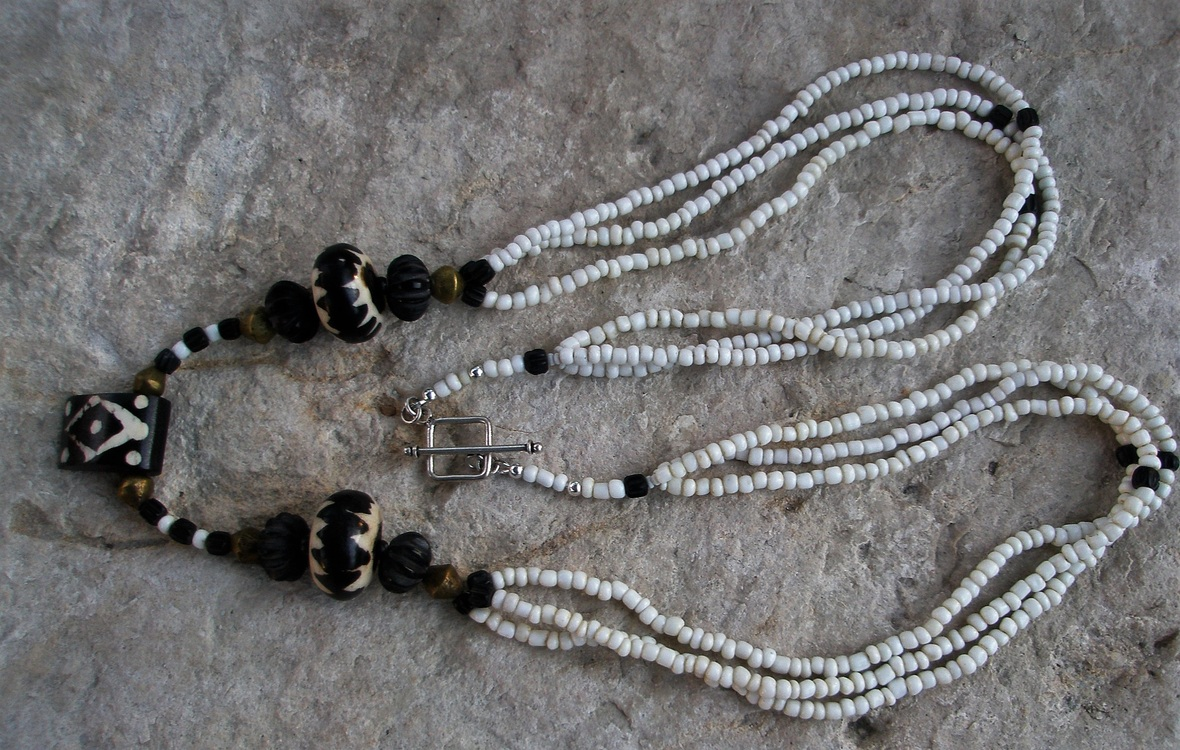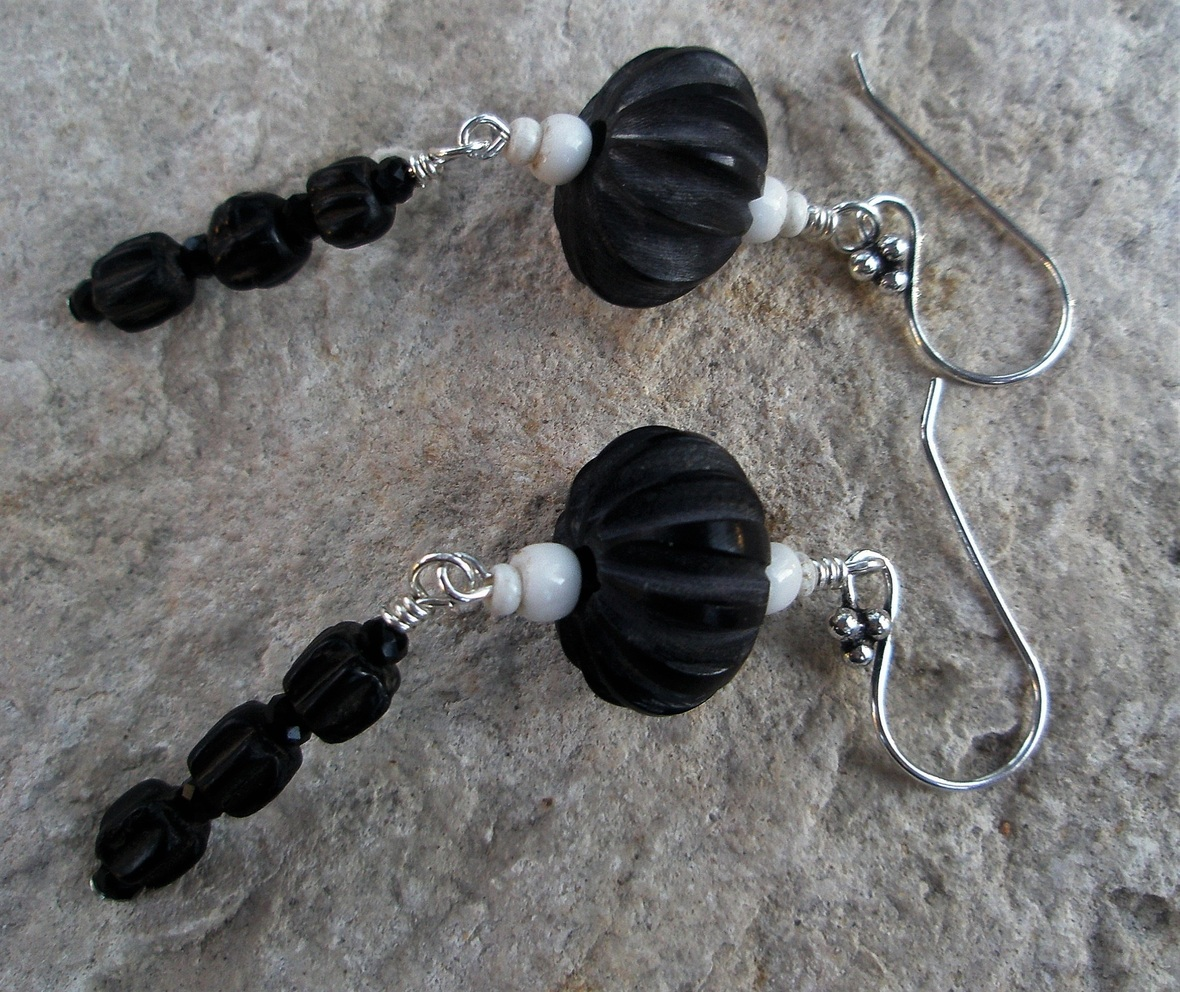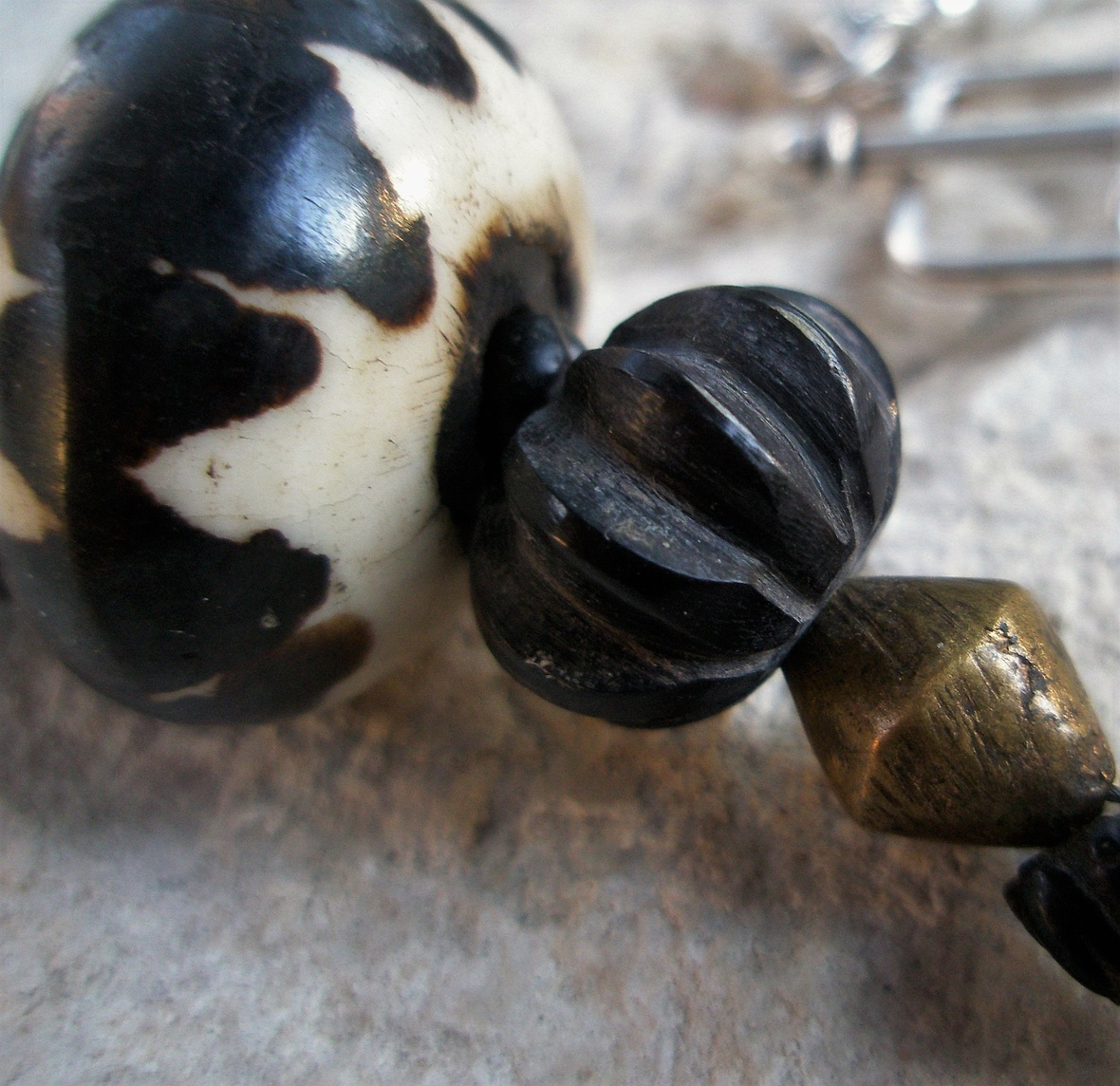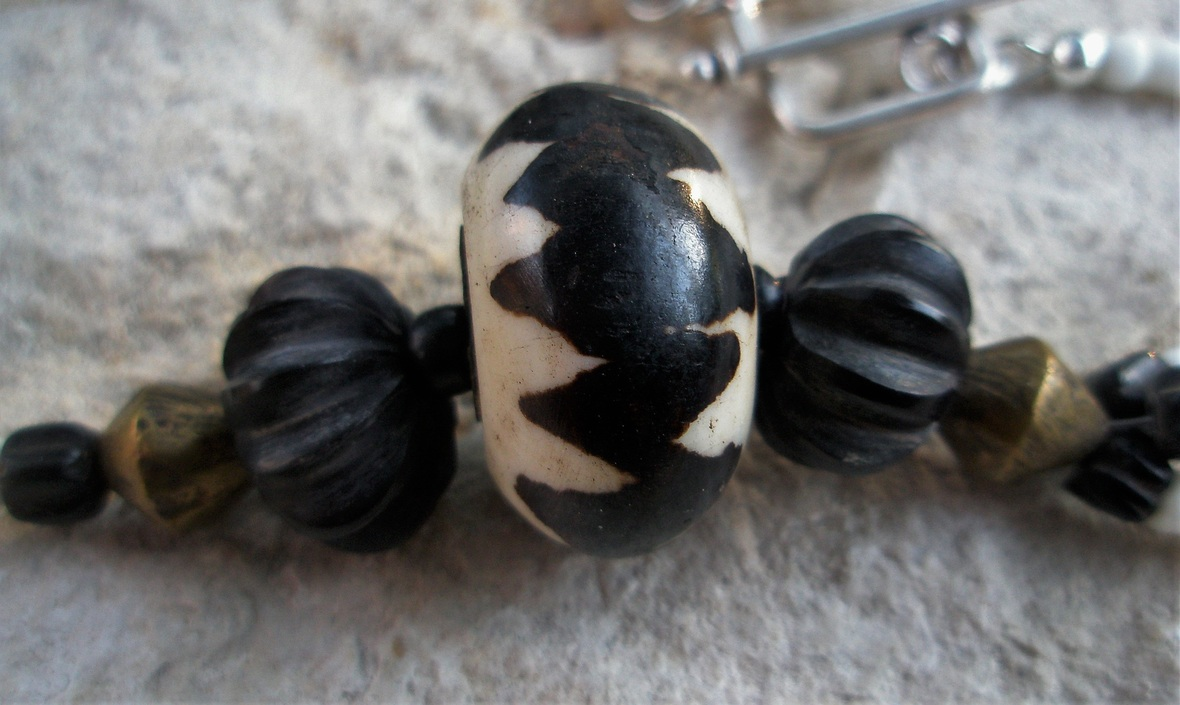table div table+table+table+table+table+table+table+table+table+table+table+table+table+table+table+table+table+table+table+table+table+table+table+table+table+table+table+table+table+table+table+table+table+table+table+table+table div table{width:100%;padding:0}table div table+table+table+table+table+table+table+table+table+table+table+table+table+table+table+table+table+table+table+table+table+table+table+table+table+table+table+table+table+table+table+table+table+table+table+table+table div table img{width:96.23%;padding:0;float:none}table div table+table+table+table+table+table+table+table+table+table+table+table+table+table+table+table+table+table+table+table+table+table+table+table+table+table+table+table+table+table+table+table+table+table+table+table+table div table td{width:100%;padding:0 1.88% 18px}/* styles *//* styles */ Continuous strand of dyed freshwater pearls with the hand cut open Jade squares. All metal is Sterling Silver. \$175.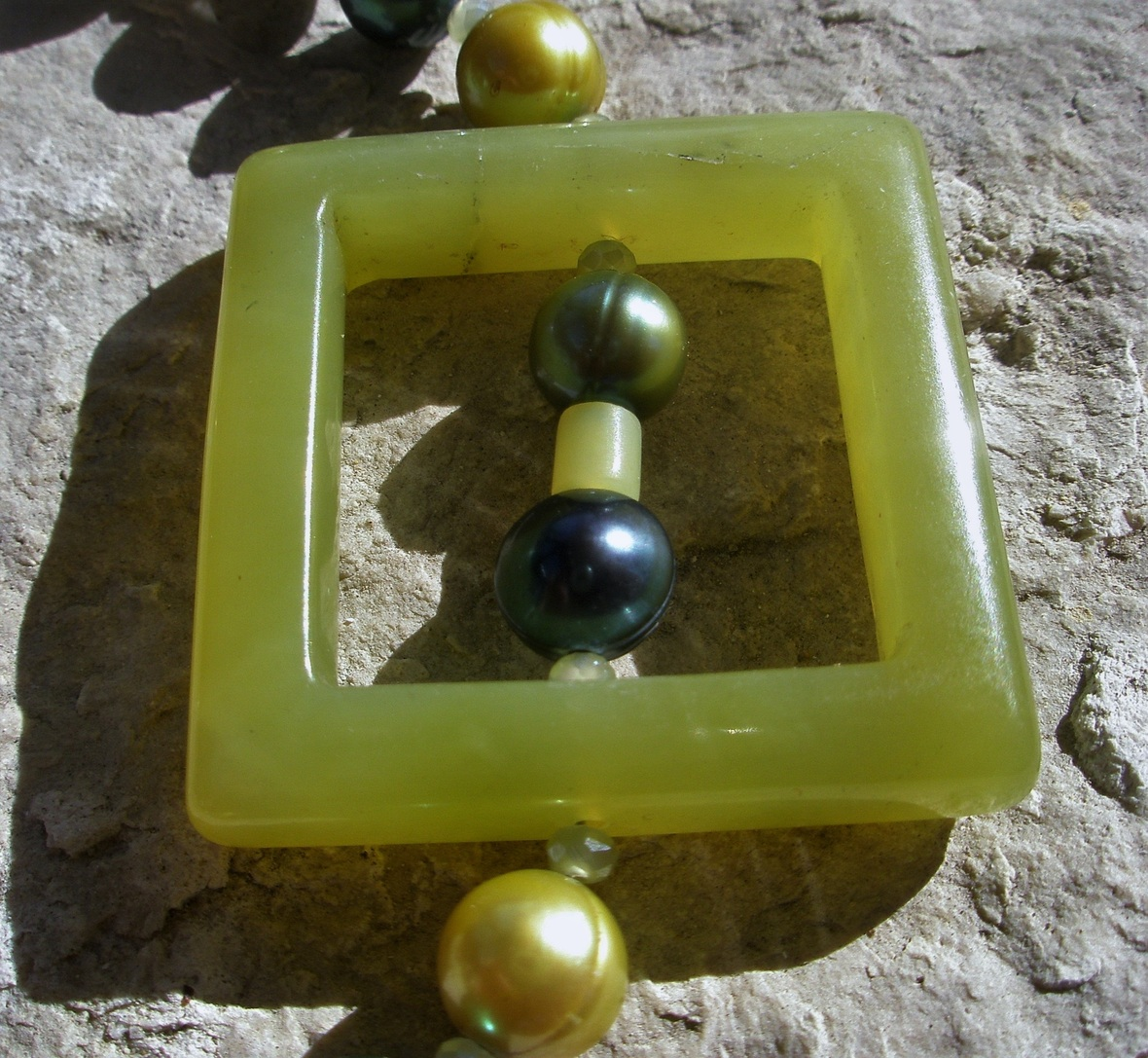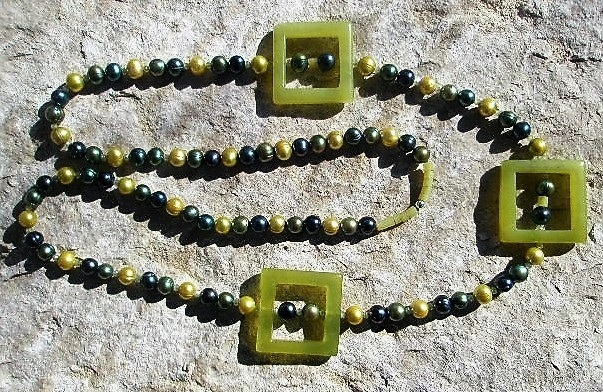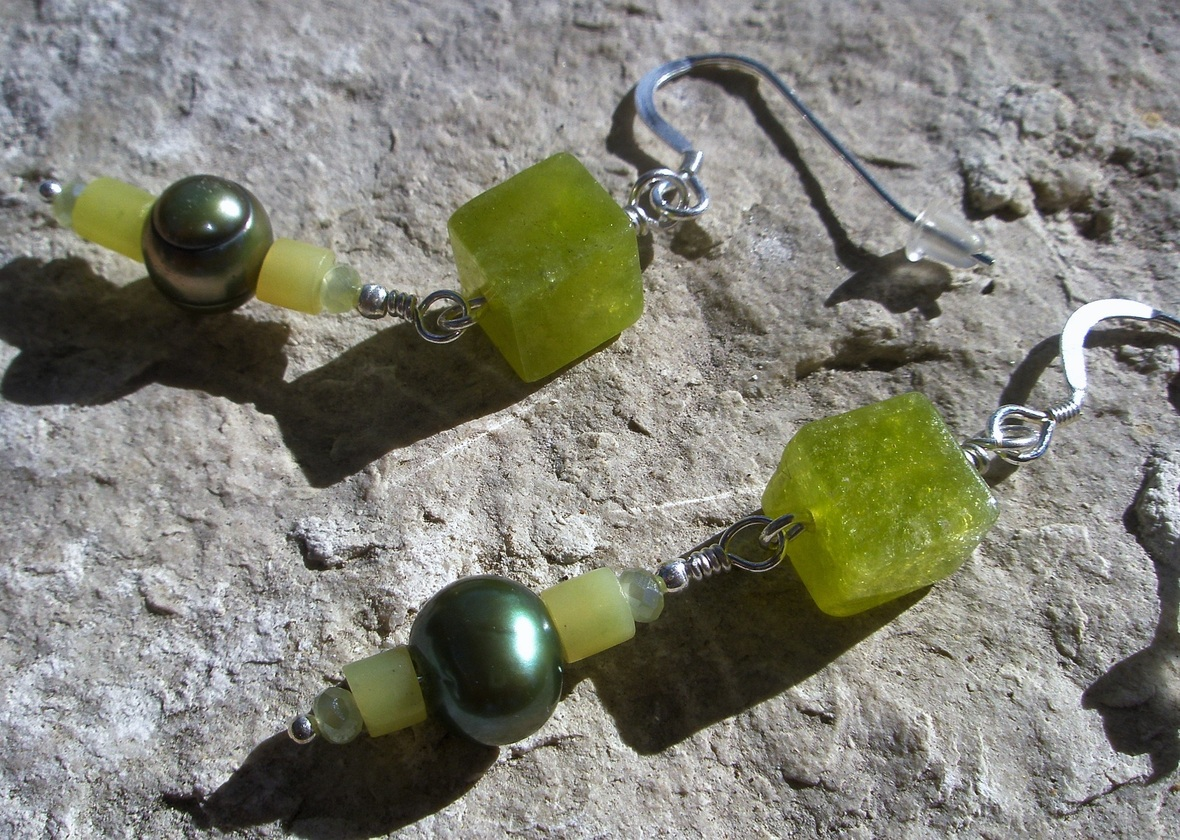table div table+table+table+table+table+table+table+table+table+table+table+table+table+table+table+table+table+table+table+table+table+table+table+table+table+table+table+table+table+table+table+table+table+table+table+table+table+table+table+table+table+table div table{width:100%;padding:0}table div table+table+table+table+table+table+table+table+table+table+table+table+table+table+table+table+table+table+table+table+table+table+table+table+table+table+table+table+table+table+table+table+table+table+table+table+table+table+table+table+table+table div table img{width:96.23%;padding:0;float:none}table div table+table+table+table+table+table+table+table+table+table+table+table+table+table+table+table+table+table+table+table+table+table+table+table+table+table+table+table+table+table+table+table+table+table+table+table+table+table+table+table+table+table div table td{width:100%;padding:0 1.88% 18px}/* styles *//* styles */ The Valentine necklace has a cast Sterling Silver heart pendant with amethyst, agate, titanium coated druzy agate, charoite, and freshwater pearls. \$175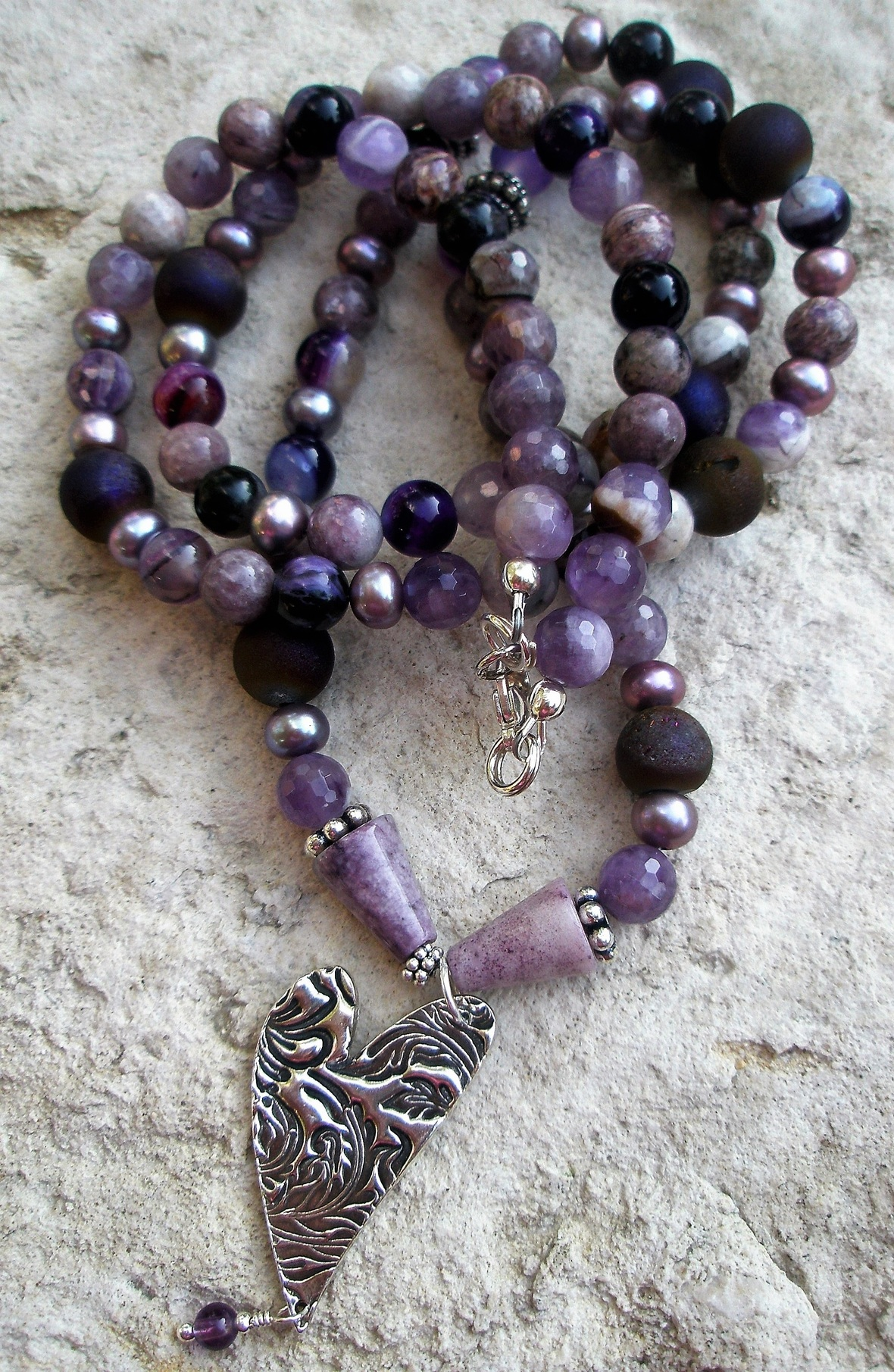table div table+table+table+table+table+table+table+table+table+table+table+table+table+table+table+table+table+table+table+table+table+table+table+table+table+table+table+table+table+table+table+table+table+table+table+table+table+table+table+table+table+table+table+table+table+table div table{width:100%;padding:0}table div table+table+table+table+table+table+table+table+table+table+table+table+table+table+table+table+table+table+table+table+table+table+table+table+table+table+table+table+table+table+table+table+table+table+table+table+table+table+table+table+table+table+table+table+table+table div table img{width:96.23%;padding:0;float:none}table div table+table+table+table+table+table+table+table+table+table+table+table+table+table+table+table+table+table+table+table+table+table+table+table+table+table+table+table+table+table+table+table+table+table+table+table+table+table+table+table+table+table+table+table+table+table div table td{width:100%;padding:0 1.88% 18px}/* styles *//* styles */ enjoy the exotic color of African Jade, Chrysoprase, Chrome Diopside, and Bronzeite. hte necklace and earrings are \$175.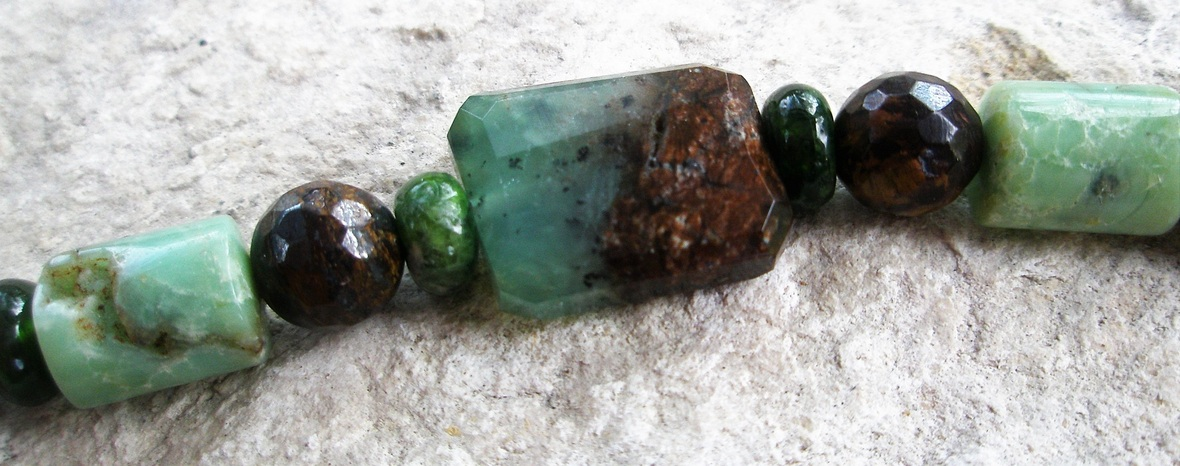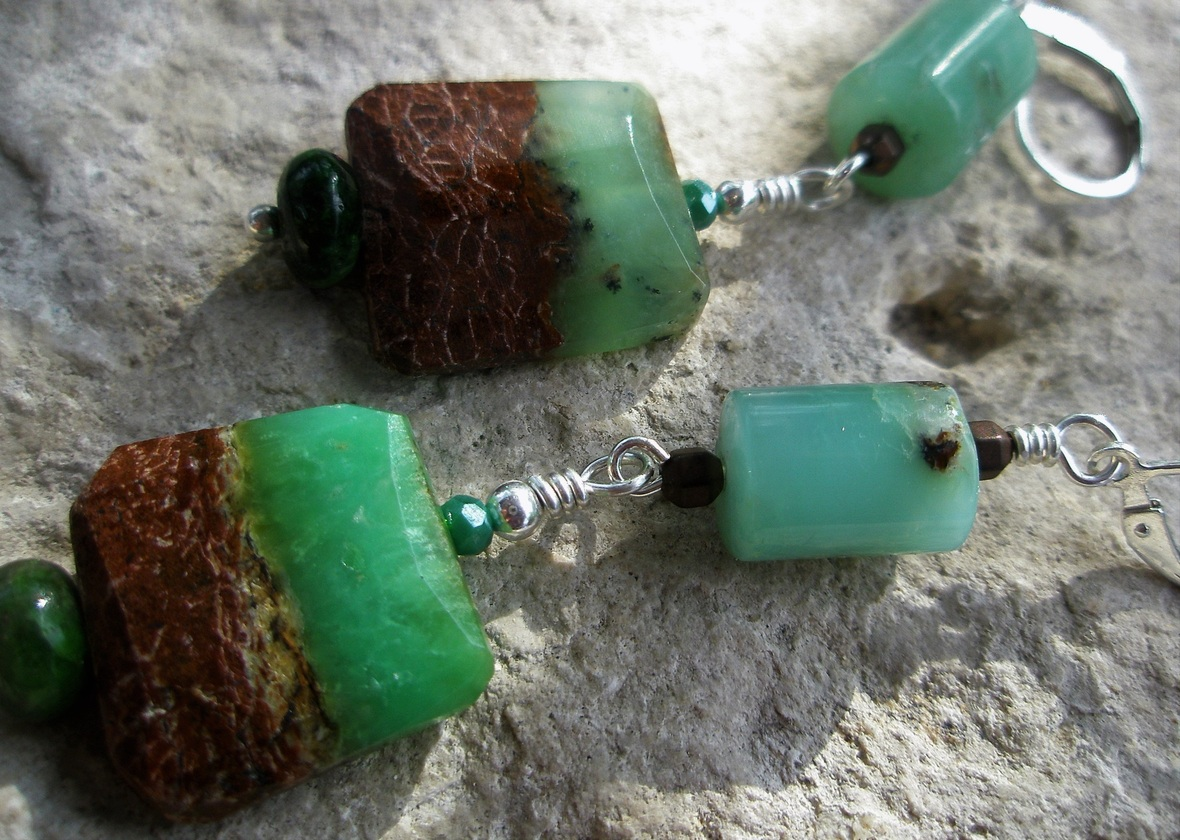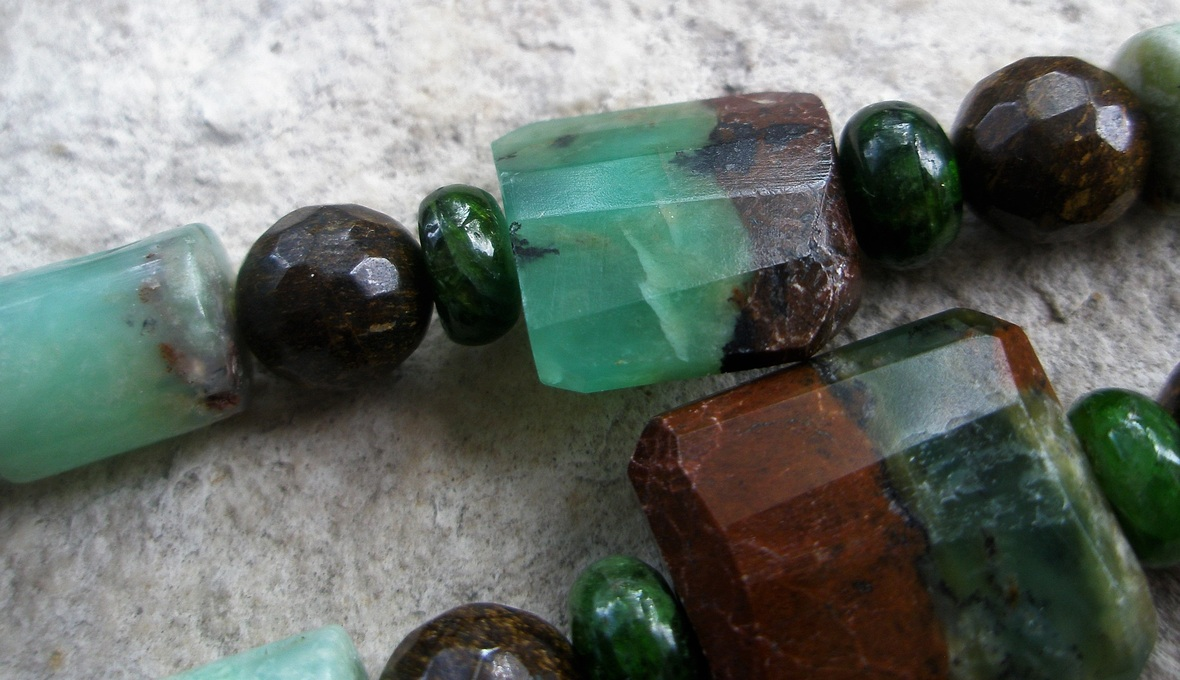/* styles */ Marta Stafford Fine Art 200 Main Street Marble Falls, TX 78657 830.693.9999 http://MartaStaffordFineArt.com